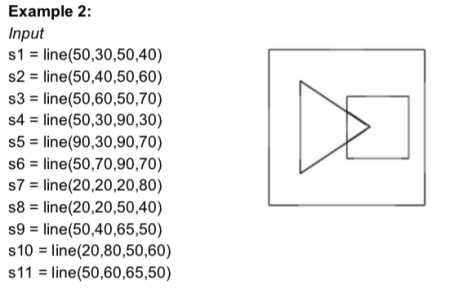Search
• remyg

# Analogical Reasoning Tests (Part One)

## Intro

Ever see those picture problems, where you have a picture relating two shapes to each other, and then have to find a matching relationship, usually with different shapes?Where words fail, pictures suffice

These are analogical reasoning tests, they posed a significant hurdle to symbolic artificial intelligence, as well as babies and reasonably intelligent animals trying to pry food from researchers, until the 1960's - and longer for the babies and animals. The goal is to identify the relationship between the first two pictures, A and B in our case, and identify the analogous relationship between two other pictures, C and K1-K3 in our case.

I was assigned a programming project to do just that.

## Test1 - Example

Now in Test 1, I have to identify the shapes and the relations between them in Picture A, and compare these identifications with Picture B. I would argue, there are only two shapes in Picture A, a rectangle and a triangle, and the triangle is to the right of the rectangle. In Picture B, there are also two shapes, a rectangle and triangle, but this time, the triangle is inside the rectangle. I would then identify the relationship between Picture A and Picture B is that the triangle was moved inside the rectangle, or that the triangle was moved to the left.

So far, I have identified the relationship, the shape on the right side is moved to left, until it is inside the shape in the center.

Now all I have to do is apply the analogous relationship to Picture C, and see which answer from K1, K2, and K3 satisfies the relationship. First, I identify the shapes in Picture C, a larger rectangle and a smaller rectangle. Now I know I must apply the analogous relationship from Picture A and B to Picture C and K1-K3. This means I must move the "smaller" rectangle to the left, until it is inside the larger rectangle in the center. Thus the answer is K1.

In other words, in Test 1: A is to B as C is to K1.

I'll let you know, writing a program to do this problem took me two months.

## Identifying Shapes is Hard

While it's simple for us humans to identify the shape and position of an object in space, programming a computer to do it is a little more difficult. In fact, there had to be some ground rules about what shapes can be made, and how my program would even take shapes as input.

The assignment specified input as four different primitive features, lines, circles, and dots. A line was identified with a marker, s, and 4 numbers following s. These 4 numbers represented the starting x and y position, and then the final x and y position AKA a line that connects the two points in 2D space. A circle had a marker, c, and 3 following numbers, representing the center of the circle in 2D space, and its radius. A dot, marker d, was followed by just 2 numbers, representing its position in 2D space (x and y again).

Two important rules to follow:

1. No lines overlap, in other words, I can't make two shapes from one line.

2. Lines connect only at starting and ending points, I don't have to worry about making shapes from lines intersecting each other.

So, I had to identify each shape by connecting lines, and identifying circles and dots.Program input and its diagram

I found shapes by following the starting and ending points of each line, and if there was that connected to one its points, I would add that line to the current shape until it reached its initial starting point - a completely closed shape. I'm abstracting away how to iterate through each line and find out if it meets another, and if they make a shape - I don't want to give answers out, it's not *as* simple as it sounds, but not unreasonably difficult.

The next problem was identify the relations of the shapes. I needed to figure out if the shapes were above, below, left of, right of, or inside of other shapes. In order to find the location of the shapes, I just calculated the center of the shape - or center of mass, assuming an equal distribution of mass. I determined the size of the shape by calculating the area of the shape. Combining the center and size of the shapes, I just had to perform simple comparisons to figure out where shape 1 was compared to shape 2.

So, the output of my program should be something like this:So easy, a hungry monkey could do it

Note: the program identifies the shape, the primitive features that make up the shape, and each shape's relation to one another. The relations, identified with relation(shape1,shape2), are read as: [shape1] is [relation] [shape2].

There you have it, the first month of programming finished. 3 weeks were spent on identifying shapes and the relations each had to one another.

## Closing Remarks

Probably the most difficult part of this problem is figuring out how to identify shapes from many connecting lines. Outside of Test1, I only provided images with clearly defined shapes, each group of lines were separated from one another. Permuting all possible combinations of shapes can be much more difficult, even for human brains. I'll leave you with an example - try to see how many different combinations of shapes you can make!

Remember the rules:

1. No lines overlap, in other words, I can't make two shapes from one line.

2. Lines connect only at starting and ending points, I don't have to worry about making shapes from lines intersecting each other.